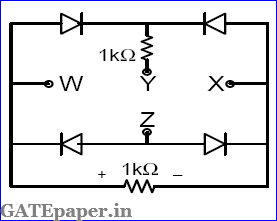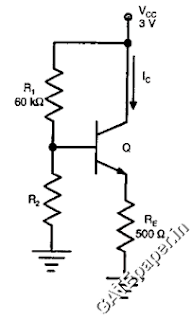### GATE 2013 ECE Video Solutions on Analog Circuits (Analog Electronics)

1. In the circuit shown below, what is the output voltage (Vout), if a silicon transistor Q and an ideal OP-AMP are used?a. - 15 volts
b. - 0.7 volts
c. + 0.7 volts
d. + 15 volts

2. In a voltage – voltage feedback as shown below, which one of the following statements is TRUE, if the gain K is increased?a. The input impedance increases and output impedance decreases
b. The input impedance increases and output impedance increases
c. The input impedance decreases and output impedance decreases
d. The input impedance decreases and output impedance increases

3. The small signal resistance (ie. dVB/dID) in KΩ offered by the n – channel MOSFET M shown in the figure below, at a bias point of VB = 2 volts is (device data for M: device Transconductance parameter KN = µNCOX(W/L) = 40 µA/V2, threshold voltage VTN = 1 volt, and neglecting body effect and channel length modulation effects)a. 12.5
b. 25
c. 50
d. 100

4. The ac schematic of an NMOS common source stage is shown in the figure below, where part of the biasing circuit has been omitted for simplicity. For the n – channel MOSFET M, the Transconductance gm = 1 mA/V and body effect and channel length modulation effect are to be neglected. The lower cutoff frequency in Hz of the circuit approximately ata. 8
b. 32
c. 50
d. 200

5. In the circuit shown below, the OP-AMPs are ideal. Then Vout in volts isa. 4
b. 6
c. 8
d. 10

6. A voltage 1000sinωt volts is applied across YZ. Assuming ideal diodes, the voltage measured across WX in volts isa. Sinωt
b. (Sinωt + |Sinωt|) / 2
c. (Sinωt - |Sinωt|) / 2
d. 0 for all t

7. In the circuit shown below, the knee current of ideal zener diode is 10 mA. To maintain 5 volts across the load RL, the minimum value of RL in Ω’s and the minimum power rating of the zener diode in mW respectively area. 125 and 125
b. 125 and 250
c. 250 and 125
d. 250 and 250

8. In the circuit shown below, the silicon NPN transistor Q has a very high value of β. The required value of R2 in KΩ to produce IC = 1 mA isa. 20
b. 30
c. 40
d. 50